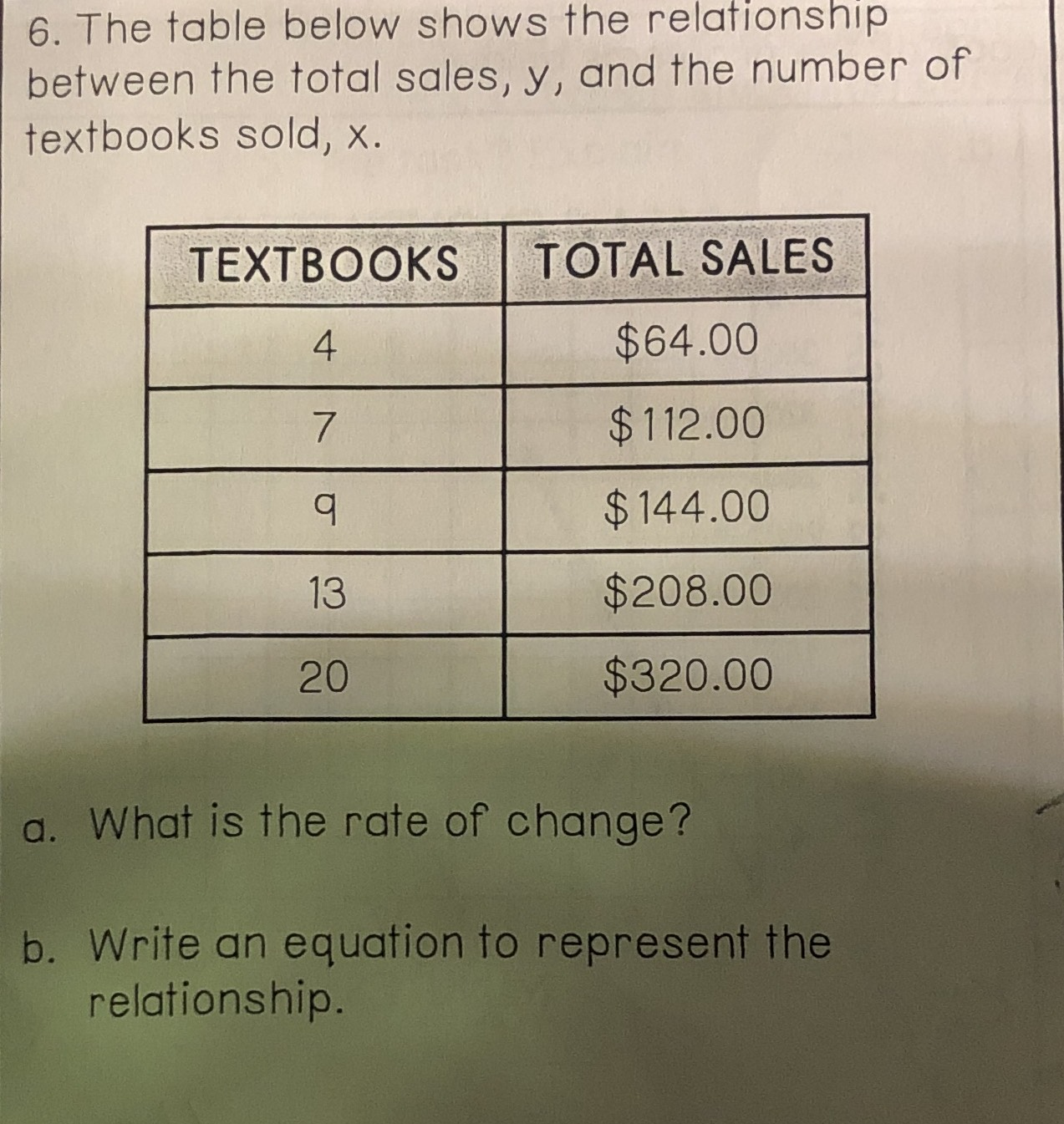### ¿Todavía tienes preguntas de matemáticas?

Pregunte a nuestros tutores expertos
Algebra
Pregunta6. The table below shows the relationship between the total sales, $$y$$ , and the number of textbooks sold, $$x$$ .

a. What is the rate of change?

b. Write an equation to represent the relationship.